# An introduction to FGLMtrunc

## Introduction

FGLMtrunc is a package that fits truncated Functional Generalized Linear Models as described in Liu, Divani, and Petersen (2020). It implements methods for both functional linear and functional logistic regression models. The solution path is computed efficiently using active set algorithm with warm start. Optimal smoothing and truncation parameters ($$\lambda_s, \lambda_t$$) are chosen by Bayesian information criterion (BIC).

To install FGLMtrunc directly from CRAN, type in R console this command:

install.packages("FGLMtrunc")

To load the FGLMtrunc package, type in R console:

library(FGLMtrunc)

The function for fitting model is fglm_trunc, which have arguments to customize the fit. Below are details on some required arguments:

• X.curves is required for matrix of functional predictors.

• Y is required for response vector.

• Either nbasis or knots is needed to define the interior knots of B-spline.

Please use ?fglm_trunc for more details on function arguments. We will demonstrate usages of other commonly used arguments by examples.

## Functional Linear Regression (family="gaussian")

Functional linear regression model is the default choice of function fglm_trunc with argument family="gaussian". For illustration, we use dataset LinearExample, which we created beforehand following Case I in simulation studies section from Liu et. al. (2020). This dataset contains $$n=200$$ observations, and functional predictors are observed at $$p=101$$ timepoints on $$[0,1]$$ interval. The true truncation point is $$\delta = 0.54$$.

data(LinearExample)
Y_linear = LinearExample$Y Xcurves_linear = LinearExample$X.curves
timeGrid = seq(0, 1, length.out = 101)
plot(timeGrid, LinearExample$beta.true, type = 'l', main = 'True coefficient function', xlab = "t", ylab=expression(beta(t)))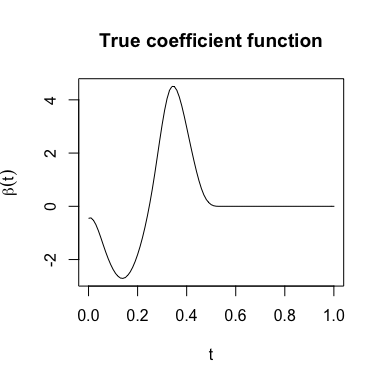### Fitting FGLMtrunc model for linear regression We fit the model using 50 B-spline basis with default degree=3 for cubic splines. Since argument grid is not specified, an equally spaced sequence of length $$p=101$$ on $$[0,1]$$ interval (including boundaries) will automatically be used. fit = fglm_trunc(Y_linear, Xcurves_linear, nbasis = 50) fglm_trunc also supports parallel computing to speed up the running time of tuning regularization parameters. Parallel backend must be registered before hand. Here is an example of using parallel with doMC backend (we cannot run the code here since it is not available for Windows) : library(doMC) registerDoMC(cores = 2) fit = fglm_trunc(Y_linear, Xcurves_linear, nbasis = 50, parallel = TRUE) One can also manually provides grid or knots sequences (or both). If knots is specified, nbasis will be ignored. k <- 50 - 3 - 1 #Numbers of knots = nbasis - degree - 1 knots_n <- seq(0, 1, length.out = k+2)[-c(1, k+2)] # Remove boundary knots fit2 = fglm_trunc(Y_linear, Xcurves_linear, grid = timeGrid, knots = knots_n) fit and fit2 fitted models will have the same results. fit is an object of class FGLMtrunc that contains relevant estimation results. Please use ?fglm_trunc for more details on function outputs. Function call and truncation point will be printed with print function: print(fit) #> #> Call: fglm_trunc(Y = Y_linear, X.curves = Xcurves_linear, nbasis = 50) #> #> #> Optimal truncation point: 0.52 ### Plotting with fitted FGLMtrunc model We can visualize the estimates of functional parameter $$\beta$$ directly with plot: plot(fit)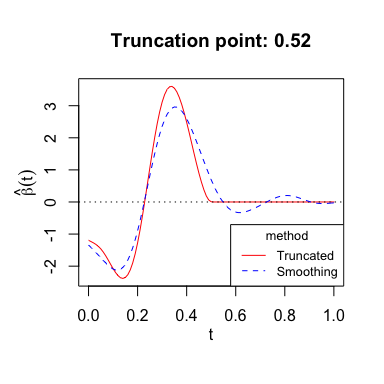#> NULL The plot shows both smoothing and truncated estimates of $$\beta$$. We can set argument include_smooth=FALSE to show only truncated estimate. ### Predicting with fitted FGLMtrunc model Predict method for FGLMtrunc fits works similar to predict.glm. Type "link" is the default choice for FGLMtrunc object. For linear regression, both type "link" and "response" return fitted values. newX.curves is required for these predictions. predict(fit, newX.curves = Xcurves_linear[1:5,]) #> [,1] #> [1,] 2.1337413 #> [2,] 1.2286605 #> [3,] 1.8207552 #> [4,] -0.2430198 #> [5,] 0.5901628 To get truncated estimate of $$\beta$$, we can use either fit$beta.truncated or predict function:

predict(fit, type = "coefficients")
#>    -1.194642e+00 -1.233189e+00 -1.274041e+00 -1.321971e+00 -1.382684e+00
#>    -1.461724e+00 -1.560226e+00 -1.676778e+00 -1.806592e+00 -1.942359e+00
#>   -2.075460e+00 -2.195993e+00 -2.293871e+00 -2.358836e+00 -2.381285e+00
#>   -2.352969e+00 -2.266606e+00 -2.117256e+00 -1.901351e+00 -1.619372e+00
#>   -1.273650e+00 -8.713600e-01 -4.219470e-01  6.139018e-02  5.633530e-01
#>    1.068248e+00  1.560120e+00  2.023704e+00  2.444542e+00  2.810437e+00
#>    3.112588e+00  3.344368e+00  3.503214e+00  3.588157e+00  3.601536e+00
#>    3.546803e+00  3.429575e+00  3.256279e+00  3.034685e+00  2.773190e+00
#>    2.481013e+00  2.167832e+00  1.843081e+00  1.516008e+00  1.196723e+00
#>    8.962979e-01  6.237543e-01  3.847519e-01  1.897238e-01  6.071026e-02
#>    8.314897e-03  1.796018e-06  0.000000e+00  0.000000e+00  0.000000e+00
#>    0.000000e+00  0.000000e+00  0.000000e+00  0.000000e+00  0.000000e+00
....

## Functional Logistic Regression (family="binomial")

For logistic regression, we use dataset LogisticExample, which is similar to LinearExample, but the response $$Y$$ was generated as Bernoulli random variable.

data(LogisticExample)
Y_logistic = LogisticExample$Y Xcurves_logistic = LogisticExample$X.curves

### Fitting FGLMtrunc model for logistic regression

Similarly, we fit the model using 50 B-spline basis with default choice of cubic splines. We need to set family="binomial" for logistic regression. Printing and plotting are the same as before.

fit4 = fglm_trunc(Y_logistic, Xcurves_logistic, family="binomial", nbasis = 50)
print(fit4)
#>
#> Call:  fglm_trunc(Y = Y_logistic, X.curves = Xcurves_logistic, family = "binomial", nbasis = 50)
#>
#>
#> Optimal truncation point: 0.58
plot(fit4)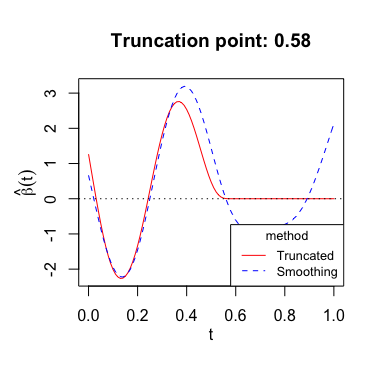#> NULL

### Predicting with fitted FGLMtrunc model for logistic regression

For functional logistic regression, each type option returns a different prediction:

• type="link" gives the linear predictors which are log-odds.

• type="response" gives the predicted probabilities.

• type="coefficients" gives truncated estimate of functional parameter $$\beta$$ as before.

logistic_link_pred = predict(fit4, newX.curves = Xcurves_logistic, type="link")
plot(logistic_link_pred, ylab="log-odds")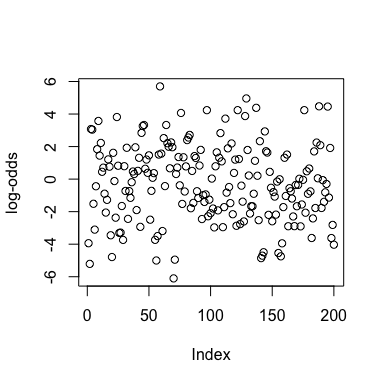logistic_response_pred = predict(fit4, newX.curves = Xcurves_logistic, type="response")
plot(logistic_response_pred, ylab="predicted probabilities")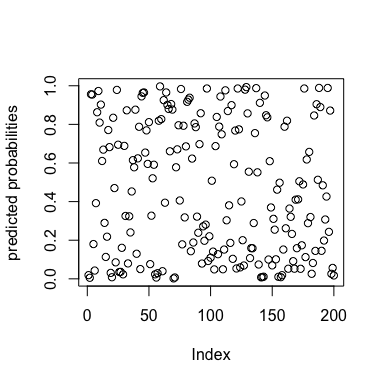## Functional Linear Regression with scalar predictors

### Fitting FGLMtrunc model

FGLMtrunc allows using scalar predictors together with functional predictors. First, we randomly generate observations for scalar predictors:

scalar_coef <- c(1, -1, 0.5) # True coefficients for scalar predictors
set.seed(1234)
S <- cbind(matrix(rnorm(400), nrow=200), rbinom(200, 1, 0.5))  # Randomly generated observations for scalar predictors. Binary coded as 0 and 1.
colnames(S) <- c("s1", "s2", "s3")

Next, we modify the response vector from LinearExample so that it takes into account scalar predictors:

Y_scalar <- Y_linear + (S %*% scalar_coef)

Then we fit FGLMtrunc model with the matrix of scalar predictors S:

fit_scalar = fglm_trunc(X.curves=Xcurves_linear, Y=Y_scalar, S=S, nbasis = 50)
fit_scalar
#>
#> Call:  fglm_trunc(Y = Y_scalar, X.curves = Xcurves_linear, S = S, nbasis = 50)
#>
#>  Intercept      s1      s2     s3
#>     1.0865  1.0937 -1.0411 0.4233
#>
#> Optimal truncation point: 0.49

Fitted coefficients for scalar predictors are close to the true values.

### Predicting with scalar predictors

To make prediction with fitted model using scalar predictors, we need to specified argument newS:

predict(fit_scalar, newX.curves = Xcurves_linear[1:5,], newS=S[1:5,])
#>            [,1]
#> [1,]  0.3837103
#> [2,]  1.2553910
#> [3,]  3.2764746
#> [4,] -3.4882131
#> [5,]  0.7946992